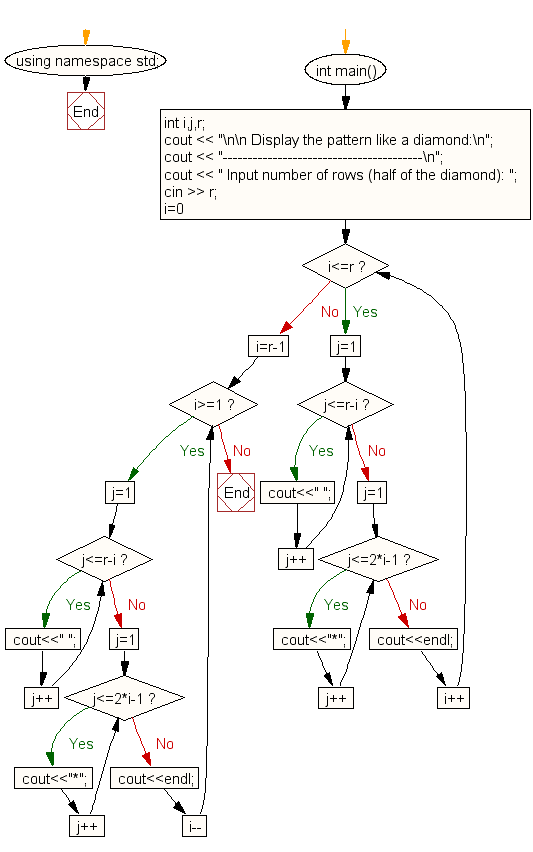﻿ C++ Exercises: Display the pattern like a diamond - w3resource# C++ Exercises: Display the pattern like a diamond

## C++ For Loop: Exercise-44 with Solution

Write a program in C++ to display the pattern like a diamond.

Sample Solution:-

C++ Code :

``````#include <iostream>
using namespace std;

int main()
{
int i,j,r;
cout << "\n\n Display the pattern like a diamond:\n";
cout << "----------------------------------------\n";
cout << " Input number of rows (half of the diamond): ";
cin >> r;
for(i=0;i<=r;i++)
{
for(j=1;j<=r-i;j++)
cout<<" ";
for(j=1;j<=2*i-1;j++)
cout<<"*";
cout<<endl;
}
for(i=r-1;i>=1;i--)
{
for(j=1;j<=r-i;j++)
cout<<" ";
for(j=1;j<=2*i-1;j++)
cout<<"*";
cout<<endl;;
}
}
``````

Sample Output:

``` Display the pattern like a diamond:
----------------------------------------
Input number of rows (half of the diamond): 5

*
***
*****
*******
*********
*******
*****
***
*
```

Flowchart:C++ Code Editor:

Contribute your code and comments through Disqus.

What is the difficulty level of this exercise?

﻿

## C++ Programming: Tips of the Day

What is a smart pointer and when should I use one?

This answer is rather old, and so describes what was 'good' at the time, which was smart pointers provided by the Boost library. Since C++11, the standard library has provided sufficient smart pointers types, and so you should favour the use of std::unique_ptr, std::shared_ptr and std::weak_ptr.

There was also std::auto_ptr. It was very much like a scoped pointer, except that it also had the "special" dangerous ability to be copied - which also unexpectedly transfers ownership.

It was deprecated in C++11 and removed in C++17, so you shouldn't use it.

```std::auto_ptr<MyObject> p1 (new MyObject());
std::auto_ptr<MyObject> p2 = p1; // Copy and transfer ownership.
// p1 gets set to empty!
p2->DoSomething(); // Works.
p1->DoSomething(); // Oh oh. Hopefully raises some NULL pointer exception.
```

Ref : https://bit.ly/3mc9GHE